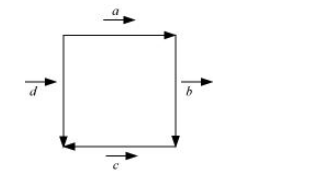# In Figure, identify the following vectors.

Question:

In Figure, identify the following vectors.(i) Coinitial

(ii) Equal

(iii) Collinear but not equal

Solution:

(i) Vectors $\vec{a}$ and $\vec{d}$ are coinitial because they have the same initial point.

(ii) Vectors $\vec{b}$ and $\vec{d}$ are equal because they have the same magnitude and direction.

(iii) Vectors $\vec{a}$ and $\vec{c}$ are collinear but not equal. This is because although they are parallel, their directions are not the same.